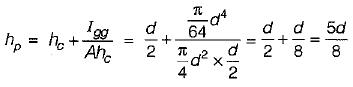Courses

# Hydrostatic Force On Surface

## 10 Questions MCQ Test Mock Test Series for Civil Engineering (CE) GATE 2020 | Hydrostatic Force On Surface

Description
This mock test of Hydrostatic Force On Surface for Civil Engineering (CE) helps you for every Civil Engineering (CE) entrance exam. This contains 10 Multiple Choice Questions for Civil Engineering (CE) Hydrostatic Force On Surface (mcq) to study with solutions a complete question bank. The solved questions answers in this Hydrostatic Force On Surface quiz give you a good mix of easy questions and tough questions. Civil Engineering (CE) students definitely take this Hydrostatic Force On Surface exercise for a better result in the exam. You can find other Hydrostatic Force On Surface extra questions, long questions & short questions for Civil Engineering (CE) on EduRev as well by searching above.
QUESTION: 1

Solution:
QUESTION: 2

### When the water surface coincides with the top edge of a rectangular vertical gate 40 m (wide) x 3 m (deep), then the depth of centre of pressure is

Solution:

Depth of centre of pressure,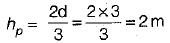QUESTION: 3

### In which of the following arrangements would the vertical force in the cylinder due to water be maximum

Solution:

Vertical force will be maximum when the cylinder is completely submerged in water.

QUESTION: 4

An isosceles triangular plate of base 3 m and altitude 3 m is immersed vertically in an oil of specific gravity 0.8. The base of the plate coincides with the free surface of oil. The centre of pressure will lie at a distance of (from free surface)

Solution: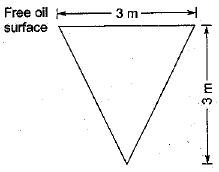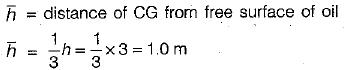Depth of pressure (h*) from free oil surface is given by,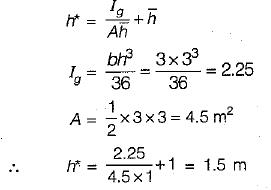QUESTION: 5

A vertically immersed surface of size b x d has its width b parallel to the water surface and depth of its centroid from the water surface is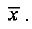The distance of its centre of water pressure from the water surface is

Solution:

Centre of water pressure,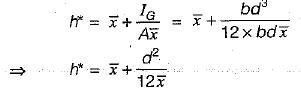QUESTION: 6

For a vertical semicircular plate submerged in a homogeneous liquid with its diameter ‘d' at the free surface, the depth of centre of pressure from the free surface is

Solution: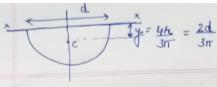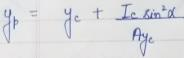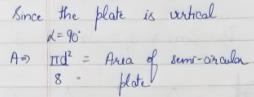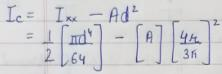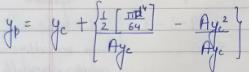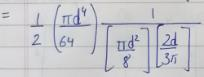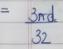QUESTION: 7

The position of centre of pressure on a plane surface immersed vertically in a static mass of fluid is

Solution:
QUESTION: 8

A vertical rectangular plane surface is submerged in water such that its top and bottom surfaces are 1.5 m and 6.0 m respectively below the free surface. The position of centre of pressure below the free surface will be at a distance of

Solution: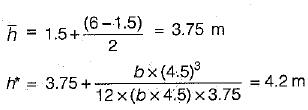QUESTION: 9

The horizontal component of force on a curved surface is equal to the

Solution:
QUESTION: 10

A circular plate of diameter d is submerged in water vertically, so that the topmost point is just at the water surface. The centre of pressure on the plate will be below the water surface at a depth of

Solution: